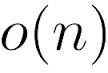bs/// Sublinear Algorithms for Big Datasets

#Sublinear Algorithms for Big Datasets# Team

• Grigory Yaroslavtsev grigory (at) grigory.us
• Brilliant Teaching Assistants from the University of Buenos Aires. Want to become one? Send me an e-mail.

# Sketch* ;)

Increasingly large datasets are being collected by governments, private companies and research institutions. This motivates increased interest in the design and analysis of algorithms for rigorous analysis of such data. In many cases, even linear space/time algorithms can be too slow. Therefore, there has been growing body of work on "sub-linear algorithms", that use space or time that are sub-linear in the input size. In this course we will cover such algorithms, which can be used for the analysis of distributions, graphs, data streams and high-dimensional real-valued data.

We focus on two types of sublinear algorithms: sub-linear time algorithms, and sketching/streaming algorithms. The former access only a small number of input elements and perform very limited computation; the answer is then inferred using approaches such as random sampling or property testing. The latter extract only a small amount of information about the input (a "sketch") and compute an answer based on that. Manifestation of this approach include data stream algorithms and randomized dimensionality reduction.

This course is partially based on the Sublinear Algorithms class by Piotr Indyk and Ronitt Rubinfeld at MIT, the Big Data class by Jealni Nelson at Harvard and the Sublinear Algorithms class by Sofya Raskhodnikova at Penn State. It also includes some recent work by the lecturer.

* Usually there is an abstract here.

# Lectures

• Introduction. (Slides: [pptx], [pdf]).
Probability basics. Introduction to streaming algorithms, Moriss's algorithm.
• Sketching and streaming algorithms I. (Slides: [pptx], [pdf]).
Approximating the median. AMS-sketching. Frequency moments via AMS. F2-moment via 4-wise independent hashing. Distinct elements. Count-Min sketch.
• Sketching and streaming algorithms II. (Slides: [pptx], [pdf], Graph sketching (by Andrew McGregor): [pdf]).
Count-Min sketch (continued), Count-Sketch. L2-sampling. L0-sampling. L1 sparse recovery. Graph sketching.
• Sublinear-time algorithms I. (Slides: [pptx], [pdf], Sublinear-time algoirthms (by Sofya Raskhodnikova): [pdf]).
Dimension reduction, Johnson-Lindenstrauss transform. Introduction to sublinear-time algorithms: models, approximation, property testing. Basic examples: testing images, sortedness, connectedness.
• Sublinear-time algorithms II + Introduction to MapReduce. (Slides: MapReduce [pptx], [pdf], Lp-testing [pptm],[pdf], Sublinear-time algoirthms (by Sofya Raskhodnikova): [pdf]).
Approximating weight of the Minimum Spanning Tree. Testing properties of real-valued functions (Lp-testing). MapReduce algorithms, Euclidean Minimum Spanning Tree.

# Bibliography

• Noga Alon, Yossi Matias, Mario Szegedy: The Space Complexity of Approximating the Frequency Moments. J. Comput. Syst. Sci. 58(1): 137-147 (1999)
• Broder, Andrei Z. (1997), "On the resemblance and containment of documents", Compression and Complexity of Sequences: Proceedings, Positano, Amalfitan Coast, Salerno, Italy, June 11-13, 1997, IEEE, pp. 21–29
• Johnson, William B.; Lindenstrauss, Joram (1984), "Extensions of Lipschitz mappings into a Hilbert space", Conference in Modern Analysis and Probability (New Haven, Conn., 1982), Contemporary Mathematics 26, Providence, RI: American Mathematical Society, pp. 189–206
• Funda Ergün, Sampath Kannan, Ravi Kumar, Ronitt Rubinfeld, Mahesh Viswanathan, Spot-Checkers. Journal of Computer System and Sciences 2000, STOC 1998.
• Oded Goldreich, Dana Ron, Property testing in bounded degree graphs. Algorithmica 2002, STOC 1997.
• Bernard Chazelle, Ronitt Rubinfeld, Luca Trevisan, Approximating the Minimum Spanning Tree Weight in Sublinear Time. SIAM Journal of Computing 2005, ICALP 2001.
• Noga Alon, Eldar Fischer, Michael Krivelevich, Mario Szegedy, Efficient testing of large graphs, Combinatorica 20 (2000), 451-476.
• Oded Goldreich, Dan Ron, Approximating average parameters of graphs, Random Struct. Algorithms 32(4): 473-493 (2008)
• Piotr Berman, Sofya Raskhodnikova, and Grigory Yaroslavtsev, Testing properties with respect to Lp distances, STOC 2014.
• Alexandr Andoni, Aleksandar Nikolov, Krzysztof Onak, Grigory Yaroslavtsev, Parallel algorithms for geometric graph problems. STOC 2014.
• Graham Cormode, S. Muthukrishnan: An Improved Data Stream Summary: The Count-Min Sketch and Its Applications. LATIN 2004: 29-38